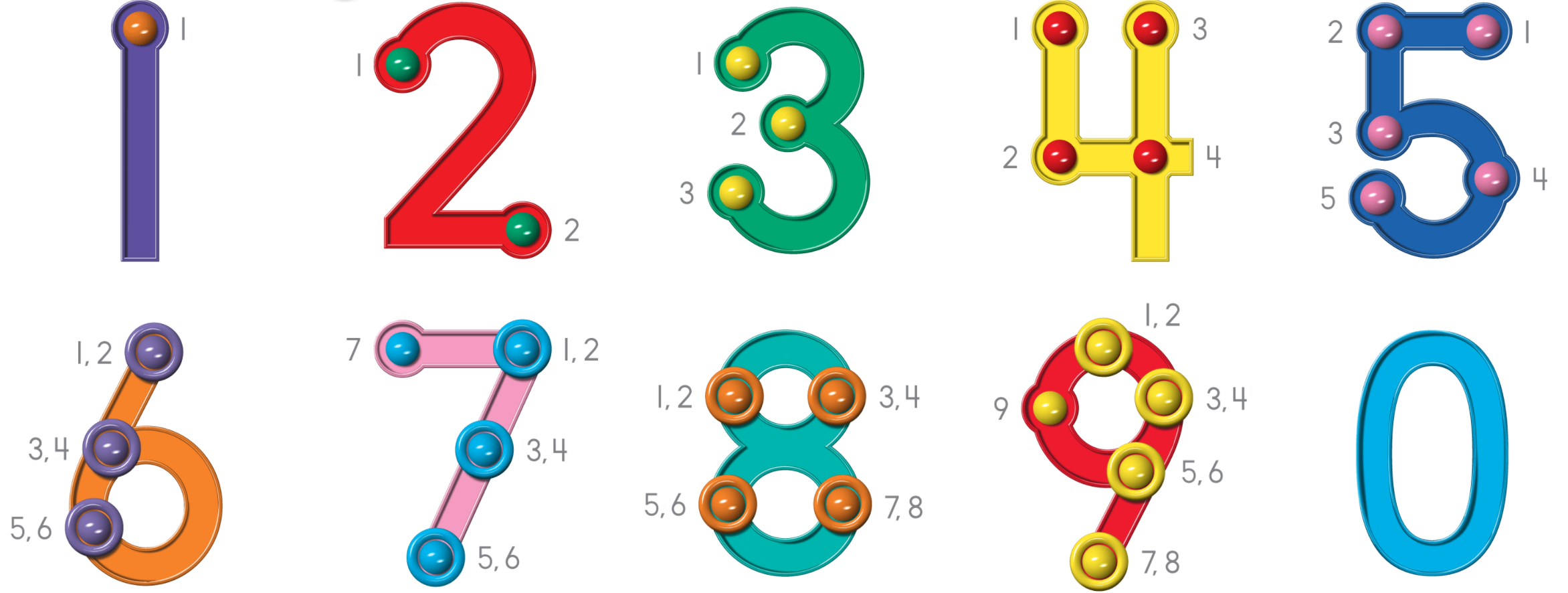# Blog# Let’s Talk About Touch Math

Touch math is a method of teaching math that involves using physical movements, such as touching or tapping, to represent mathematical concepts and perform calculations. While it has been marketed as a way to help students with learning disabilities or difficulty with math, there is limited research on its effectiveness and some concerns about its potential negative effects.

As teachers we’ve witnessed some of touch math’s long-term impacts listed here:

1. Limited transferability: Touch math relies heavily on physical manipulation and memorization, rather than abstract thinking and understanding of mathematical concepts. This means that students may struggle to apply the skills they learn with touch math to other areas of math, or to solve math problems in different formats.  Ex: skip counting, multiplication facts
2. Dependence on a specific method: Students who are taught math using touch math may become reliant on the specific techniques and physical movements used in this method, and may struggle to adapt to other methods of solving math problems.
3. Misconceptions about math: Touch math can promote the idea that math is about following a set of specific rules and procedures, rather than understanding and applying mathematical concepts. This can lead to a lack of understanding and confidence in math, and may make it more difficult for students to grasp more complex math concepts in the future.
4. Limited problem-solving skills: Touch math may not encourage students to think critically and creatively about math problems, which can limit their ability to solve math problems on their own and may lead to a lack of problem-solving skills.

Overall, while touch math may be useful for some students in the short term, there are concerns about its potential negative long-term effects on math learning and problem-solving skills. It is important for educators to carefully consider the benefits and drawbacks of touch math and to use a variety of teaching methods and approaches to ensure that students are receiving a well-rounded math education.